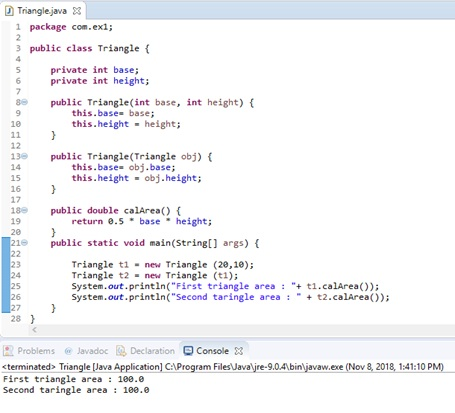# What is the Difference Between Copy Constructor and Assignment Operator

The main difference between copy constructor and assignment operator is that copy constructor is a type of constructor that helps to create a copy of an already existing object without affecting the values of the original object while assignment operator is an operator that helps to assign a new value to a variable in the program.

A constructor is a special method that helps to initialize an object when creating it. It has the same name as the class name and has no return type. A programmer can write a constructor to give initial values to the instance variables in the class. If there is no constructor in the program, the default constructor will be called. Copy constructor is a type of constructor that helps to create a copy of an existing object. On the other hand, assignment operator helps to assign a new value to a variable.

### Key Areas Covered

1. What is Copy Constructor
– Definition, Functionality
2. What is Assignment Operator
– Definition, Functionality
3. What is the Difference Between Copy Constructor and Assignment Operator
– Comparison of Key Differences

### Key Terms

Constructor, Copy Constructor, Assignment Operator, Variable## What is Copy Constructor

In programming, sometimes it is necessary to create a separate copy of an object without affecting the original object. Copy constructor is useful in these situations. It allows creating a replication of an existing object of the same class. Refer the below example.Figure 1: Program with copy constructor

The class Triangle has two instance variables called base and height. In line 8, there is a parameterized constructor. It takes two arguments. These values are assigned to the instance variables base and height. In line 13, there is a copy constructor. It takes an argument of type Triangle. New object’s base value is assigned to the instance variable base. Similarly, the new object’s height value is assigned to the instance variable height. Furthermore, there is a method called calArea to calculate and return area.

In the main method, t1 and t2 are Triangle objects. The object t1 is passed when creating the t2 object. The copy constructor is called to create t2 object. Therefore, the base and the height of the t2 object is the same as the base and height of t1 object. Finally, both objects have the same area.

## What is Assignment Operator

An assignment operator is useful to assign a new value to a variable. The assignment operator is “=”.  When there is a statement as c = a + b; the summation of ‘a’ and ‘b’ is assigned to the variable ‘c’.Figure 2: Program with assignment operator

The class Number has an instance variable called num. There is a no parameter constructor in line 7. However, there is a parameterized constructor in line 9. It takes an argument and assigns it to the instance variable using the assignment operator. In line 12, there is a method called display to display the number. In the main method, num1 and num2 are two objects of type Number. Printing num1 and num2 gives the references to those objects. The num3 is of type Number. In line 24, num1 is assigned to num3 using the assignment operator. Therefore, num3 is referring to num1 object. So, printing num3 gives the num1 reference.

The assignment operator and its variations are as follows.

 Operator Functionality Example = Assigns the right operand to the left operand z = x +y += Add the right operand to the left operand and assign the result to the left operand z += y is equivalent to z = z +y – = Subtract the right operand from the left operand and assign the result to left operand. z -= y is equivalent to z = z -y * = Multiply the right operand with the left operand and assign the result to left operand. z *=y is equivalent to z = z*y /= Divides the left operand with right operand and assigns the answer to left operand. z / = y is equivalent to z = z/y %= Takes the modulus of two operands and assigns the answer to left operand. z % = y is equivalent to z = z % y <<= Left shift AND assignment operator z << 5 is equivalent to  z= z <<5 >>= Right shift AND assignment operator z >>5 is equivalent to  z= z>>5 &= Bitwise AND assignment operator z&=5 is equivalent to z = z&5 ^= Bitwise exclusive OR and assignment operator z ^=5 is equivalent to z = z^5 |= Bitwise inclusive OR and assignment operator z |= 5 is equivalent to z = z|5

## Difference Between Copy Constructor and Assignment Operator

### Definition

Copy constructor is a special constructor for creating a new object as a copy of an existing object. In contrast, assignment operator is an operator that is used to assign a new value to a variable. These definitions explain the basic difference between copy constructor and assignment operator.

### Functionality with Objects

Functionality with objects is also a major difference between copy constructor and assignment operator. Copy constructor initializes the new object with an already existing object while assignment operator assigns the value of one object to another object which is already in existence.

### Usage

Copy constructor helps to create a copy of an existing object while assignment operator helps to assign a new value to a variable. This is another difference between copy constructor and assignment operator.

### Conclusion

The difference between copy constructor and assignment operator is that copy constructor is a type of constructor that helps to create a copy of an already existing object without affecting the values of the original object while assignment operator is an operator that helps to assign a new value to a variable in the program.

##### Reference:

1. Thakur, Dinesh. “Copy Constructor in Java Example.” Computer Notes, Available here.